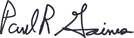Cart

# Positive Interference with Mercury 202

Bob uses an HP 4500 and noticed that tungsten at M186 could cause a positive interference with mercury 202. He asked Paul for a good correction equation to reliably counter this effect.

Dear Bob,

The interference is due to the molecular oxide of W186 O16 = 202 mass. Have you tried to minimize the interference by cutting back on the sample (nebulizer) Ar flow? To use a correction equation for this type of interference would require an MO mass different from 202 where WO can be measured. The problem is that all of these masses have an Hg line present. If there was a 'clean' WO mass to measure, then the calculation for the interference correction would be simple.

In addition, interference corrections can be unsuitable in cases where the intensity of the interference is equal to or greater than the intensity of the analyte mass. In this case, the only fix I know of is to go to the Hg 201 mass, provided the WO interference can't be reduced to insignificance by reduction in the sample Ar flow.